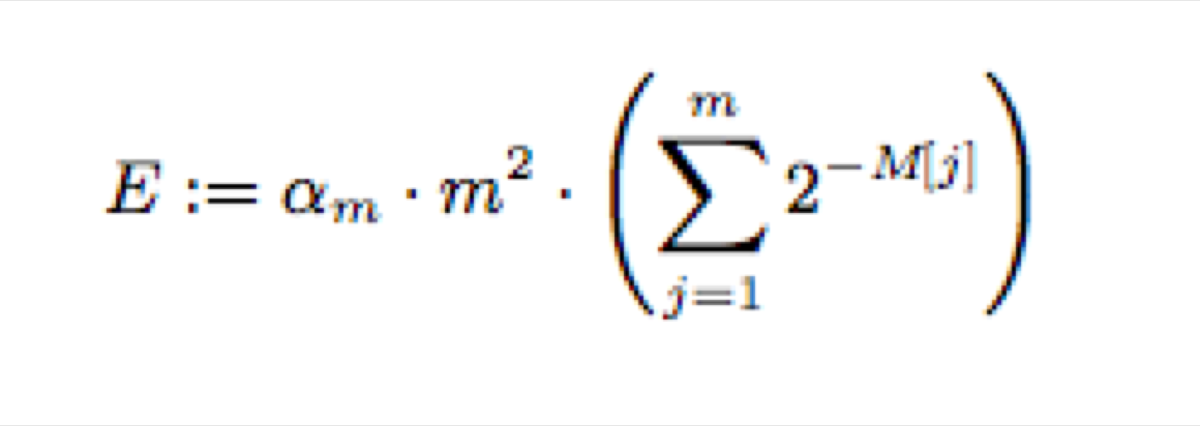I have a task for you. I send you an infinite stream of objects, and your job is to give me the number of unique elements you have seen so far. I may send you 10 elements and then ask you how many have you seen or wait until you receive 1 million elements.

There is at least one huge problem. You can add elements to a set, and when I ask you about the number of unique elements, you can give me the size of the set. Such a solution works perfectly in case of 10 elements. It may work correctly even in the case of 1 million elements, as long as you can store them somewhere.

The stream is infinite. At some point, you are going to run out of memory. Don’t be scared. I have one good news for you ;) I don’t need an exact number of unique elements, an approximation is enough for me.

You don’t need hundreds of terabytes of memory. You can accomplish that using just a few kilobytes. Hype-driven, big data developers are going to hate it ;)

# Cardinality estimation algorithm

I have a stream of squares, triangles, circles, and parallelograms. I want to count the cardinality of that stream. Obviously, the exact cardinality is equal to 4. I want to explain the concept, so don’t expect the correct result. This algorithm works well with a massive number of unique elements.

## What we need?

• HyperLogLog algorithm — probabilistic counting algorithm that estimates the number of unique elements in the given dataset

• a hashing function that accepts a shape as a parameter and returns an unsigned byte. Note that the hash values must be uniformly distributed.

• parameter m, in this case, m = 2, the number of registers

• parameters p = 1, because m = 2^p

• two bytes of memory to store the registers used by HyperLogLog, at the beginning both registres store 0.

• the bias parameter — explained at the end of this article

## Steps

1. Pass the object to the hashing function and get a hash number.

2. Convert the number to its binary representation.

3. Take the first p bite (in our example, the first bite). Use it as the identifier of the registry.

4. Pick the correct registry.

5. Take the other seven bites of the hash number, let’s call them x.

6. Calculate the number of leading zeros in the binary representation of x+1

7. Compare the number of leading zeros with the current value of the selected registry, pick the larger number.

8. Store the number calculated in step 7 as the new value of the registry.

To estimate the number of elements use the following equation:alpha_m =the bias parameter, m = number of registers, M = an array of registers so M[j] means the j-th registry

We can calculate the bias parameter using this equation:

or use the table of values from the “Probabilistic Counting Algorithms for Data Base Applications” paper by Philippe Flajolet:

In 2013, a few improvements of the algorithm have been proposed by Stefan Heule, Marc Nunkesser, and Alexander Hall in their paper: “HyperLogLog in Practice: Algorithmic Engineering of a State of The Art Cardinality Estimation Algorithm.” For example, if the number of elements is small, multiply it a little bit: m * log(m / estimated cardinality)

That is the part we need ;)

## Let’s do it with pen and paper

Now we can estimate the cardinality of the stream of shapes: